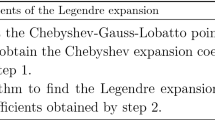# A Stabilized Galerkin Scheme for the Convection-Diffusion-Reaction Equations

## Abstract

A fully discrete stabilized scheme is proposed for solving the time-dependent convection-diffusion-reaction equations. A time derivative term results in our stabilized algorithm. The finite element method for spatial discretization and the backward Euler or Crank-Nicolson scheme for time discretization are employed. The long-time stability and convergence are established in this article. Finally, some numerical experiments are provided to confirm the theoretical analysis.

This is a preview of subscription content, log in via an institution to check access.

Price excludes VAT (USA)
Tax calculation will be finalised during checkout.

### Similar content being viewed by others### A New Algebraically Stabilized Method for Convection–Diffusion–Reaction Equations### An efficient spectral-Galerkin method for solving two-dimensional nonlinear system of advection–diffusion–reaction equations## References

1. Codina, R.: Comparison of some finite element methods for solving the diffusion-convection-reaction equation. Comput. Methods Appl. Mech. Eng. 156, 185–210 (1998)

2. Hughes, T.: Multiscale phenomena: Green’s functions, the Dirichlet-to-Neumann formulation, subgrid scale models, bubbles and the origins of stabilized methods. Comput. Methods Appl. Mech. Eng. 127, 387–401 (1995)

3. Hughes, T., Mazzei, L., Jansen, K.: Large eddy simulation and the variational multiscale method. Comput. Vis. Sci. 3, 47–59 (2000)

4. Hughes, T., Sangalli, G.: Variational multiscale analysis: the fine-scale Green’s function, projection, optimization, localization, and stabilized methods. SIAM J. Numer. Anal. 26, 1485–1503 (2007)

5. John, V., Kaya, S.: A finite element variational multiscale method for the Navier-Stokes equations. SIAM J. Sci. Comput. 26, 1485–1503 (2005)

6. John, V., Kaya, S.: Finite element error analysis of a variational multiscale method for the Navier-Stokes equations. Adv. Comput. Math. 28, 43–61 (2008)

7. John, V., Kaya, S., Layton, W.: A two-level variational multiscale method for convection-dominated convection-diffusion equations. Comput. Methods Appl. Mech. Eng. 195, 4594–4603 (2006)

8. Kaya, S., Layton, W.: Subgrid-scale eddy viscosity methods are variational multiscale methods. Technical report, University of Pittsburgh, 2003

9. Layton, W., Lee, H., Peterson, J.: A defect-correction method for the incompressible Navier-Stokes equations. Appl. Math. Comput. 129, 1–19 (2002)

10. Layton, W.: Superconvergence of finite element discretization of time relaxation models of advection. BIT Numer. Math. 47, 565–576 (2007)

11. Layton, W., Neda, M.: Truncation of scales by time relaxation. J. Math. Anal. Appl. 325, 788–807 (2007)

12. Anitescu, M., Pahlevani, F., Layton, W.: Implicit for local effects and explicit for nonlocal effects is unconditionally stable. Electron. Trans. Numer. Anal. 18, 174–187 (2004)

13. Labovsky, A., Layton, W., Manica, C., Neda, M., Rebholz, L.: The stabilized extrapolated trapezoidal finite-element method for the Navier-Stokes equations. Comput. Methods Appl. Mech. Eng. 198, 958–974 (2009)

14. Layton, W.: Introduction to the Numerical Analysis of Incompressible Viscous Flows. SIAM, Philadelphia (2008)

15. Guermond, J.: Stabilization of Galerkin approximations of transport equations by subgrid modeling. Math. Model. Numer. Anal. 33, 1293–1316 (1999)

16. Guermond, J., Marra, A., Quartapelle, L.: Subgrid stabilized projection method for 2D unsteady flows at high Reynolds numbers. Comput. Methods Appl. Mech. Eng. 195, 5857–5876 (2006)

17. Heitmann, N.: Subgridscale stabilization of time-dependent convection dominated diffusive transport. J. Math. Anal. Appl. 331, 38–50 (2007)

18. Santos, I., Almeida, R.: A nonlinear subgrid method for advection-diffusion problems. Comput. Methods Appl. Mech. Eng. 196, 4771–4778 (2007)

19. Davis, L., Pahlevani, F.: Semi-implicit schemes for transient Navier-Stokes equations and eddy viscosity models. Numer. Methods Partial Differ. Equ. 25, 212–231 (2009)

20. Hou, Y., Liu, Q.: A stabilized semi-implicit Galerkin scheme for Navier-Stokes equations. J. Comput. Appl. Math. 231, 552–560 (2009)

21. Burie, J., Marion, M.: Multilevel methods in space and time for the Navier-Stokes equations. SIAM J. Numer. Anal. 34, 1574–1599 (1997)

22. Shen, J.: Long time stability and convergence for fully discrete nonlinear Galerkin methods. Appl. Anal. 38, 201–229 (1990)

23. Heywood, J., Rannacher, R.: Finite element approximation of the nonstationary Navier-Stokes problem, part IV: error analysis for second-order time discretization. SIAM J. Numer. Anal. 27, 353–384 (1990)

24. Heywood, J., Rannacher, R.: Finite element approximation of the nonstationary Navier-Stokes problem, part II: stability of solutions and error estimates uniform in time. SIAM J. Numer. Anal. 23, 750–777 (1986)

25. Mu, M., Zhu, X.: Decoupled schemes for a non-stationary mixed Stokes-Darcy model. Math. Comput. 79(210), 707–731 (2010)

26. Bochev, P., Gunzburger, M., Shadid, J.: Stability of the SUPG finite element method for transient advection-diffusion problems. Comput. Methods Appl. Mech. Eng. 193, 2301–2323 (2004)

## Acknowledgements

The authors would like to thank the editor and the anonymous referees for their helpful comments and suggestions, which lead to substantial improvements of this presentation.

## Author information

Authors

### Corresponding author

Correspondence to Yanren Hou.

Subsidized by the Fundamental Research Funds for the Central Universities (Grant Nos. 08142013 and 08143045), NSF of China (Grant Nos. 11171269 and 11201254) and the Ph.D. Programs Foundation of Ministry of Education of China (Grant No. 20110201110027).

## Rights and permissions

Reprints and Permissions

Liu, Q., Hou, Y., Ding, L. et al. A Stabilized Galerkin Scheme for the Convection-Diffusion-Reaction Equations. Acta Appl Math 130, 115–134 (2014). https://doi.org/10.1007/s10440-013-9840-5